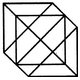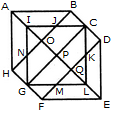# Non Verbal Reasoning - Analytical Reasoning - Discussion

Discussion Forum : Analytical Reasoning - Section 1 (Q.No. 5)
5.

Find the number of triangles in the given figure.18
20
24
27
Explanation:

The figure may be labelled as shown.The simplest triangles are IJO, BCJ, CDK, KQL, MLQ, GFM, GHN and NIO i.e. 8 in number.

The triangles composed of two components each are ABO, AHO, NIJ, IGP, ICP, DEQ, FEQ, KLM, LCP and LGP i.e.10 in number.

The triangles composed of four components each are HAB, DEF, LGI, GIC, ICL and GLC i.e. 6 in number.

Total number of triangles in the figure = 8 + 10 + 6 = 24.

Discussion:
29 comments Page 1 of 3.

Nasira farid said:   4 months ago
How to find the node numbers? Please explain.

Dinesh Kumar Shahi said:   2 years ago
Good, thanks all for explaianing.

Sharmila said:   2 years ago
@Swathi.

Here total no Of nodes are 17. But you had mentioned 14 can you please explain me how?

Lahari said:   3 years ago
How the inner nodes calculated? Please explain.

Lakshmialekhya said:   3 years ago
How the inner nodes are calculated? Please explain to me.

Ram said:   4 years ago

Girl said:   4 years ago

Swathi said:   5 years ago
@All.

The shortcut method is;

Total no.of nodes + 2(no.of inner nodes).
=14 + 2 (5),
=24.
(1)

Mukesh vaishnav said:   6 years ago
It has 25 triangles.

Ans- middle square has =8 triangles by twice method( count all triangles and then last part *2) then you see three same types of triangles at first, second and at last which has 3 - 3 - 3 equal triangles =9, and at last small and simple triangles are 8 (at sides and middles). So that's all answer is 25.

Siddharth said:   6 years ago
How to find no of nodes?